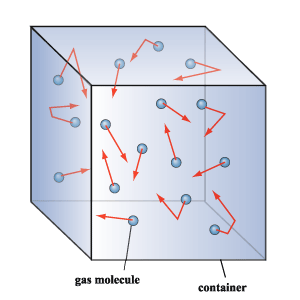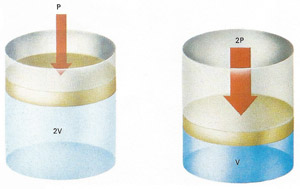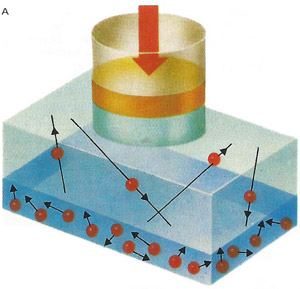A# gasThe molecules of a gas move continuously at various speeds and in various directions. Collisions with the walls of the container cause pressure and there are so many molecules in even the smallest volume that the pressure is the same everywhere in it. The actual pressure is proportional to the number of molecules in a unit volume that the pressure is the same everywhere in it. The actual pressure is proportional to the number of molecules in a unit volume and to the average kinetic energy of the molecules.When the pressure exerted by the piston is doubled, the gas volume is halved (provided temperature does not change). This is an example of Boyle's law: pressure is inversely proportional to volume.In early attempts to liquefy gases, they ere subjected to high pressure. Some gases liquefied under these conditions and a dynamic equilibrium was established between the liquid and vapor states – molecules left and entered the surface at the same rate.The experiments of Thomas Andrews (1813–85) resulted in techniques that allowed other gases to be liquefied. Pressure is increased by screwing in the plungers and transmitted through the water to the gas and air in the upper tubes. The air assumed to obey Boyle's law so that its change in volume is a measure of the pressure.Graphs of volume against pressure, called isothermals, reflect the various states of the gas. The horizontal blue line shows liquefaction and does not appear until temperature falls below 31.1°C, the critical temperature of carbon dioxide.

Gas is the state of matter in which the constituent atoms or molecules always occupy the whole of the space in which they are contained. Gases have low densities, are highly compressible over a wide range of volumes, and have no rigidity and low viscosities. A gas is distinguished from a vapor in that a gas is above the critical temperature of the substance.

A gas consists of particles that would move freely in an ideal gas, but which in a real gas are subject to small intermolecular forces known as Van der Waals forces. Gas particles move randomly at a wide range of speeds of the order of several hundred meters per second.

## Kinetic theory

The molecules of a gas are usually a large distance apart compared with their diameter and there is no regularity in their arrangement in space. Given the positions of two or three molecules, it is not possible to predict where a further one will be with any precision – the molecules are distributed at random throughout the whole volume. Gas molecules move randomly with a mean velocity comparable with that of sound, of the order of 10 m/s. Occasionally two or three of them may be found very close to one another so that their electron clouds overlap and they bind together. Such clusters are common at high pressures but they are usually short-lived.

At 0°C and a pressure of 760 mm of mercury, known as standard temperature and pressure (STP), a liter of oxygen is known to contain and 3 × 1022 (30,000 million trillion) molecules. They move with a speed of about 430 m/s (1,410 ft/s). Each oxygen molecule is 3.35 × 10-10 m across. The molecules are on average 70 × 10-10 m apart and they travel about 905 × 10-10 m between collisions; this distance is called the mean free path.

The low density of a gas can be readily understood in terms of the comparatively small number of molecules per unit volume, and the high compressibility follows from the fact that the average distance between molecules can be altered over wide limits. The lack of rigidity can be explained by the molecules being able to take up any configuration with equal ease. Further, the molecules can move long distances without encountering one another, so that there is little resistance to motion of any kind, which is the basis of the explanation of the low viscosity.

## Ideal gas equation

For a given temperature and pressure, equal volumes of gas contain the same number of molecules (2.7 × 1025 per m3 at room temperature and atmospheric pressure). The impacts of the molecules on the walls of the container are responsible for the pressure exerted by gases, which is much larger than is often appreciated: the atmosphere exerts on everything a pressure many times larger than a person's weight and without it we would simply boil and burst.

For a given mass of ideal gas, the product of the pressure (P) and the volume (V) is proportional to the absolute temperature (T):

PV = RT (the general gas law).

The constant of proportionality (R) is known as the universal gas constant and has the value 8.314 J/K-mole. The general gas law and most of the other properties of gases can be explained in terms of the kinetic theory without reference to the internal structure of the molecules.

## Boyle's law, Avogadro's principle and other outcomes of kinetic theory

As temperature rises the kinetic energy of the molecules (their energy of motion) increases by an amount that is proportional to the change in absolute temperature. In a mixture of gases the average kinetic energy becomes the same for each kind of molecule. When a gas is compressed, the volume is inversely proportional to the pressure. This relationship is known as Boyle's law, after its discoverer Robert Boyle. It exists because, when the volume is reduced, collisions with the container walls become more frequent and the pressure rises. If the temperature of a gas rises but it is not allowed to expand (its volume is held constant), the pressure again increases because molecular collisions with the walls become more forceful as well as more frequent.

Another basic gas law is called Avogadro's law (see Avogadro). It states that, at the same temperature and pressure, equal volumes of all gases contain the same number of molecules. A liter of a dense gas such as carbon dioxide contains the same number of molecules as a liter of a light gas such as hydrogen.

Gases slowly diffuse through the walls of a porous vessel because their molecules are smaller than the minute holes in the walls of the container. The rate at which they do so is inversely proportional to the square root of their density. Discovered by the British physicist Thomas Graham it is called Graham's law of diffusion. It is explained by the kinetic theory: if different gas molecules have the same average kinetic energy, the lighter ones must move more quickly. Thus light gases diffuse through pores more quickly than dense ones. More direct evidence for the kinetic theory is provided by the phenomenon of Brownian motion.